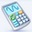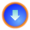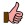Microsoft Mathematics v4.0.1108Publisher: Microsoft Corporation Size: 17.6 MB OS: Windows Total Download: 1,067 User Rating: 8.3 out of 10Category: Educational Games
Description
Microsoft Mathematics contains education tools in term of mathematical solution tools that can help students solve their school work while learning to understand step by step to get to the final result.

Microsoft Mathematics includes many features such as standard mathematical computing, equations and inequalities, trigonometry, matrix / vector, standard statistic, and an interesting feature: it can plot the equations into graphical presentation.

User Rating
Current Rating:
Excellent (10 points):
Good (5 points):